Next: Construction of hard-core predicates Up: Further results on pseudo-random Previous: Further results on pseudo-random

Hard-Core Predicates and Pseudo-Random Numbers

We have seen several functions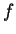with the property that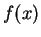was easy to compute but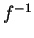was difficult. Such anis called a one-way function.16 A predicate is a property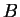which is true or false for any. Typical examples might be is an odd number'' or is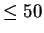.'' A hard-core predicate for a functionis a predicate such that
1.
there is an efficient algorithm for deciding whether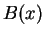is true
2.
if we are given, there is no efficient algorithm for guessing whetheris true which has a probability much greater than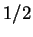of being right.
The example we used in the quadratic number generator waswhere we are looking only at thosewhich are squares.

Theorem 32   Ifis a hard-core predicate for, the random number generator in which a seedis chosen at random, giving the sequence of bitssatisfies the Next Bit Condition.

As a corollary, the sequence,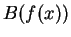,...satisfies all efficient tests, using the arguments in the preceding section.

Proof: If there were a program which took as input a se quence of bits and could guess the next bit, we could get a good guess onwithknown by asking the next-bit predictor what would occur next in the sequence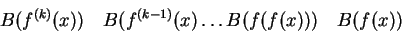(this is really the same proof as in the case of the quadratic generator).

Footnotes

... function.16
In later work, we will be defining a one-way function to be such that there is no efficiently computablewith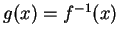for most.Next: Construction of hard-core predicates Up: Further results on pseudo-random Previous: Further results on pseudo-random
Translated from LaTeX by Scott Sutherland
1998-03-15